• 计算机图形学中的应用非常广泛的变换是一种称为仿射变换的特殊变换，在仿射变换中的基本变换包括平移、旋转、缩放、剪切这几种。本文以及接下来的几篇文章重点介绍一下关于旋转的变换，包括二维旋转变换、三维旋转...
. 简介

计算机图形学中的应用非常广泛的变换是一种称为仿射变换的特殊变换，在仿射变换中的基本变换包括平移、旋转、缩放、剪切这几种。本文以及接下来的几篇文章重点介绍一下关于旋转的变换，包括二维旋转变换、三维旋转变换以及它的一些表达方式（旋转矩阵、四元数、欧拉角等）。

2. 绕原点二维旋转

首先要明确旋转在二维中是绕着某一个点进行旋转，三维中是绕着某一个轴进行旋转。二维旋转中最简单的场景是绕着坐标原点进行的旋转，如下图所示：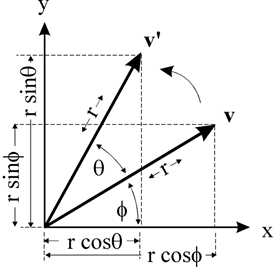如图所示点v 绕 原点旋转θ 角，得到点v’，假设 v点的坐标是(x, y) ，那么可以推导得到 v’点的坐标（x’, y’)(设原点到v的距离是r，原点到v点的向量与x轴的夹角是ϕ )x=rcosϕy=rsinϕx′=rcos(θ+ϕ)y′=rsin(θ+ϕ)
通过三角函数展开得到x′=rcosθcosϕ−rsinθsinϕy′=rsinθcosϕ+rcosθsinϕ
带入x和y表达式得到x′=xcosθ−ysinθy′=xsinθ+ycosθ
写成矩阵的形式是：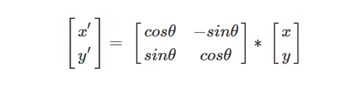尽管图示中仅仅表示的是旋转一个锐角θ的情形，但是我们推导中使用的是三角函数的基本定义来计算坐标的，因此当旋转的角度是任意角度（例如大于180度，导致v’点进入到第四象限）结论仍然是成立的。

3. 绕任意点的二维旋转

绕原点的旋转是二维旋转最基本的情况，当我们需要进行绕任意点旋转时，我们可以把这种情况转换到绕原点的旋转，思路如下：
1. 首先将旋转点移动到原点处
2. 执行如2所描述的绕原点的旋转
3. 再将旋转点移回到原来的位置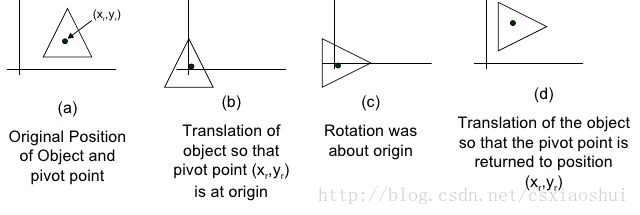也就是说在处理绕任意点旋转的情况下需要执行两次平移的操作。假设平移的矩阵是T(x,y)，也就是说我们需要得到的坐标 v’=T(x,y)*R*T(-x,-y)（我们使用的是列坐标描述点的坐标，因此是左乘，首先执行T(-x,-y)）

在计算机图形学中，为了统一将平移、旋转、缩放等用矩阵表示，需要引入齐次坐标。（假设使用2x2的矩阵，是没有办法描述平移操作的，只有引入3x3矩阵形式，才能统一描述二维中的平移、旋转、缩放操作。同理必须使用4x4的矩阵才能统一描述三维的变换）。

对于二维平移，如下图所示，P点经过x和y方向的平移到P’点，可以得到：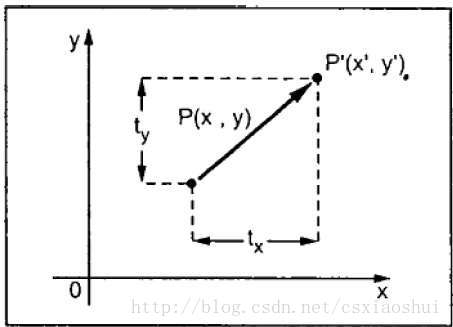x′=x+tx

y′=y+ty
由于引入了齐次坐标，在描述二维坐标的时候，使用（x，y，w）的方式（一般w=1），于是可以写成下面矩阵的形式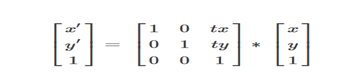按矩阵乘法展开，正好得到上面的表达式。也就是说平移矩阵是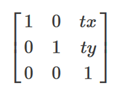如果平移值是（-tx，-ty）那么很明显平移矩阵式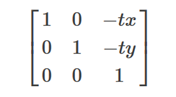我们可以把2中描述的旋转矩阵也扩展到3x3的方式，变为：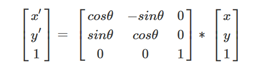从平移和旋转的矩阵可以看出，3x3矩阵的前2x2部分是和旋转相关的，第三列与平移相关。有了上面的基础之后，我们很容易得出二维中绕任意点旋转的旋转矩阵了，只需要把三个矩阵乘起来即可：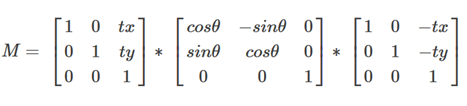4. 三维基本旋转

我们可以把一个旋转转换为绕基本坐标轴的旋转，因此有必要讨论一下绕三个坐标值x、y、z的旋转。
本文在讨论过程中使用的是类似于OpenGL中定义的右手坐标系，同时旋转角度的正负也遵循右手坐标系的约定。如下图所示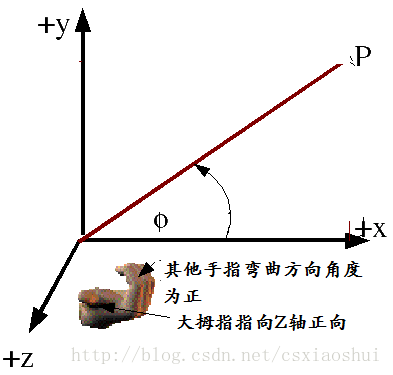4.1 绕X轴的旋转

在三维场景中，当一个点P(x,y,z)绕x轴旋转θ角得到点P’(x’,y’,z’)。由于是绕x轴进行的旋转，因此x坐标保持不变，y和z组成的yoz（o是坐标原点）平面上进行的是一个二维的旋转，可以参考上图（y轴类似于二维旋转中的x轴，z轴类似于二维旋转中的y轴），于是有：x′=xy′=ycosθ−zsinθz′=ysinθ+zcosθ
写成（4x4）矩阵的形式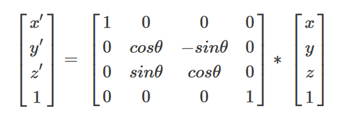4.2 绕Y轴旋转

绕Y轴的旋转和绕X轴的旋转类似，Y坐标保持不变，除Y轴之外，ZOX组成的平面进行一次二维的旋转（Z轴类似于二维旋转的X轴，X轴类似于二维旋转中的Y轴，注意这里是ZOX，而不是XOZ，观察上图中右手系的图片可以很容易了解到这一点），同样有：x′=zsinθ+xcosθy′=yz′=zcosθ−xsinθ
写成（4x4）矩阵的形式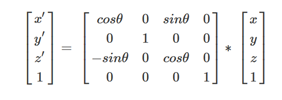4.3 绕Z轴旋转

与上面类似，绕Z轴旋转，Z坐标保持不变，xoy组成的平面内正好进行一次二维旋转（和上面讨论二维旋转的情况完全一样）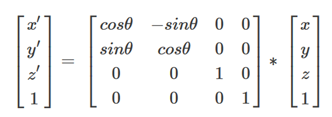4.4 小结

上面描述了三维变换中绕单一轴旋转的矩阵表达形式，绕三个轴旋转的矩阵很类似，其中绕y轴旋转的矩阵与绕x和z轴旋转的矩阵略有点不同（主要是三个轴向顺序和书写矩阵的方式不一致导致的，绕三个不同坐标旋转轴以及其他二个坐标轴组成平面的顺序是： XYZ(绕x轴） YZX（绕y轴） ZXY（绕z轴），其中绕y轴旋转，其他两个轴是ZX，这和我们书写矩阵按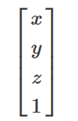的方式不一致，而导致看起来绕Y轴旋转的矩阵似乎是和其他两个矩阵不一致。如果我们颠倒写法，将公式写成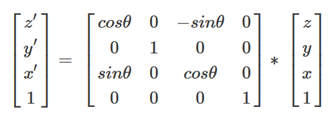的方式，那么这三个旋转矩阵看起来在形式上就统一了，都是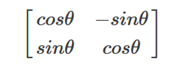这种表现形式了（左上角都是−sinθ）

5. 绕任意轴的三维旋转

绕任意轴的三维旋转可以使用类似于绕任意点的二维旋转一样，将旋转分解为一些列基本的旋转。绕任意轴旋转如下图所示：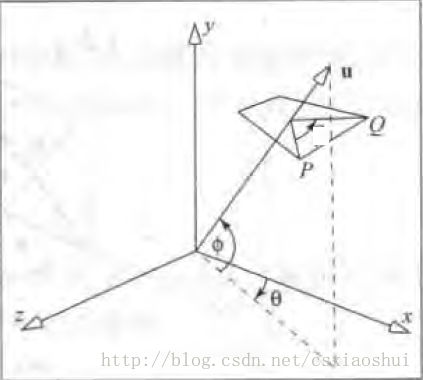P点绕向量u旋转θ角，得到点Q，已知P点的坐标和向量u，如何求Q点的坐标。
我们可以把向量u进行一些旋转，让它与z轴重合，之后旋转P到Q就作了一次绕Z轴的三维基本旋转，之后我们再执行反向的旋转，将向量u变回到它原来的方向，也就是说需要进行的操作如下：
1. 将旋转轴u绕x轴旋转至xoz平面
2. 将旋转轴u绕y轴旋转至于z轴重合
3. 绕z轴旋转θ角
4. 执行步骤2的逆过程
5. 执行步骤1的逆过程
原始的旋转轴u如下图所示：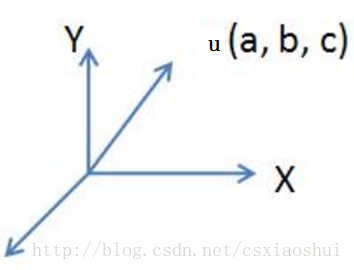第1、2、3步骤如下图所示：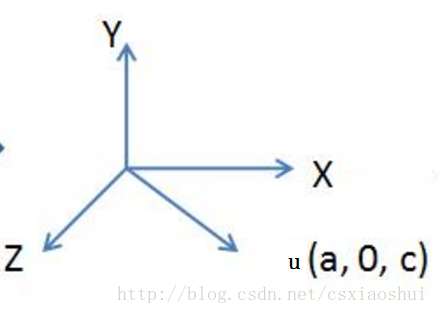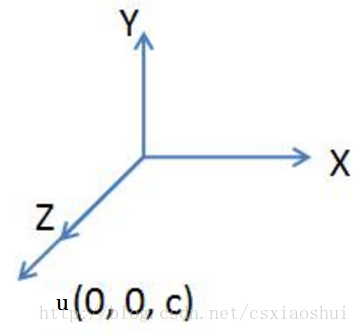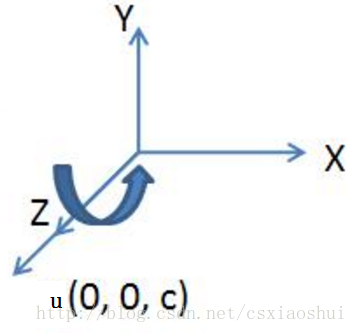步骤1将向量u旋转至xoz平面的操作是一个绕x轴的旋转操作，步骤2将向量u旋转到与z轴重合，第1、2步骤的示意图如下：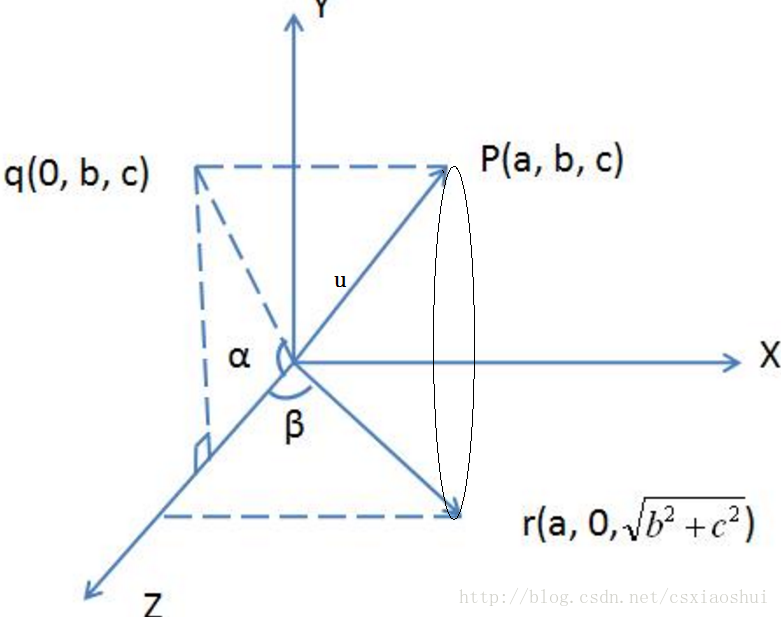作点P在yoz平面的投影点q，q的坐标是（0, b, c)，原点o与q点的连线oq和z轴的夹角就是u绕x轴旋转的角度。通过这次旋转使得u向量旋转到xoz平面（图中的or向量）【步骤1】
过r点作z轴的垂线，or与z轴的夹角为β, 这个角度就是绕Y轴旋转的角度，通过这次旋转使得u向量旋转到与z轴重合【步骤2】

步骤1中绕x轴旋转的是一次基本的绕x轴的三维旋转，按照之前的讨论，旋转矩阵是：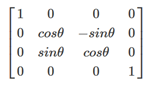这里的θ就是图中所示的α角 （注意α角度是绕x旋转的正的角度）
从图中我们还可以得到：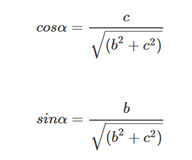于是旋转矩阵(记作 Rx(α))为：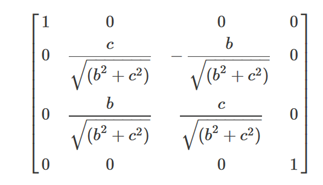在完成步骤1之后，向量u被变换到了r的位置，我们继续步骤2的操作，绕y轴旋转负的β角（注意：这里的β是负的），经过这次变换之后向量u与z轴完全重合，由于这一步也是执行的一次绕Y轴的基本旋转，旋转矩阵（记作 Ry(−β))为：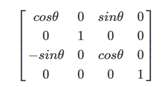使用−β替换表达式中的θ，此外根据图中描述，我们可以计算得到：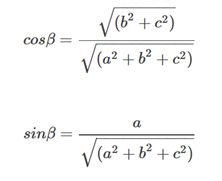带入上面的表达式，于是旋转矩阵(记作 Ry(−β))为：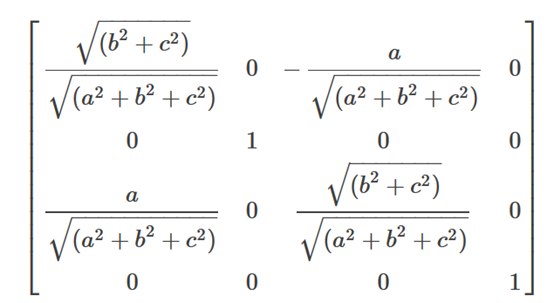在完成前面两个步骤之后，u方向和z轴完全重合，因此执行旋转θ角，执行的是一次绕z轴的基本三维旋转（记作 R(θ)，根据之前的讨论，我们可以得到：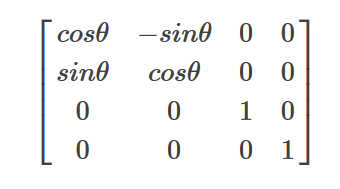最后两步骤是前面1和2的逆操作，也就是绕Y轴旋转β 和绕X轴旋转−α，这两个矩阵分别记作 Ry(β) 和 Rx(−α)，得到它们的方式很简单，只需要将上面步骤1和步骤2中的角度修改成相反数即可，也就是：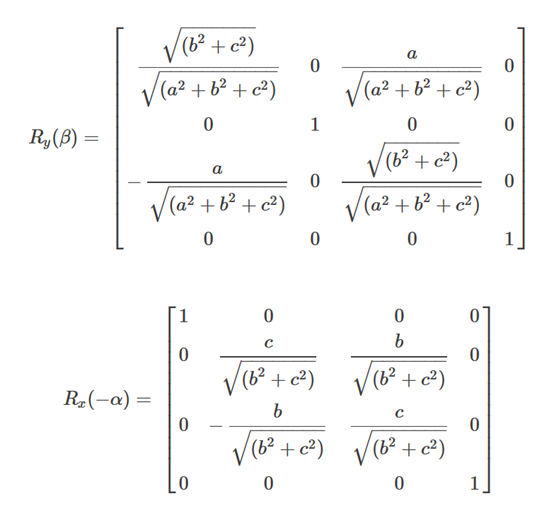最终得到 绕任意轴u旋转的旋转矩阵是【因为使用的列向量，因此执行的是左乘（从右往左）】：

MR=Rx(−α)Ry(β)Rz(θ)Ry(−β)Rx(α)=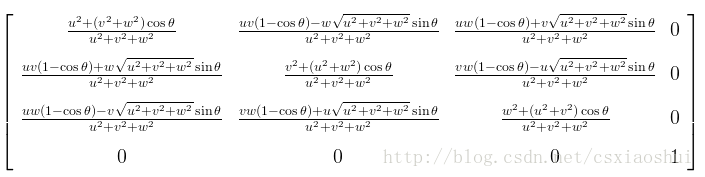(注：式中的(u,v,w)对应上文中向量(a,b,c)，公式我自己笔算过，为了减少编辑公式的时间（使用LaTex编辑太繁琐，因此找了一张公式的图片贴在此处)

如果向量是经过单位化的（单位向量），那么有a2+b2+c2=1，可以简化上述的公式，得到：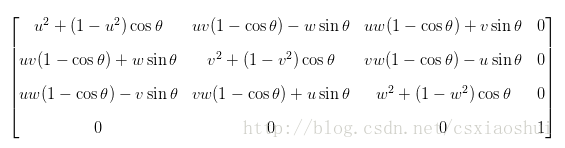6绕任意轴旋转

绕任意轴旋转的情况比较复杂，主要分为两种情况，一种是平行于坐标轴的，一种是不平行于坐标轴的，对于平行于坐标轴的，我们首先将旋转轴平移至与坐标轴重合，然后进行旋转，最后再平移回去。

将旋转轴平移至与坐标轴重合，对应平移操作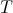旋转，对应操作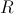步骤1的逆过程，对应操作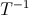整个过程就是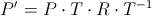对于不平行于坐标轴的，可按如下方法处理。（该方法实际上涵盖了上面的情况）

将旋转轴平移至原点
将旋转轴旋转至YOZ平面
将旋转轴旋转至于Z轴重合
绕Z轴旋转θ度
执行步骤3的逆过程
执行步骤2的逆过程
执行步骤1的逆过程
假设用v1(a1, b2, c2)和v2(a2, b2, c2)来表示旋转轴，θ表示旋转角度。为了方便推导，暂时使用右手系并使用列向量，待得出矩阵后转置一下即可，上面步骤对应的流程图如下。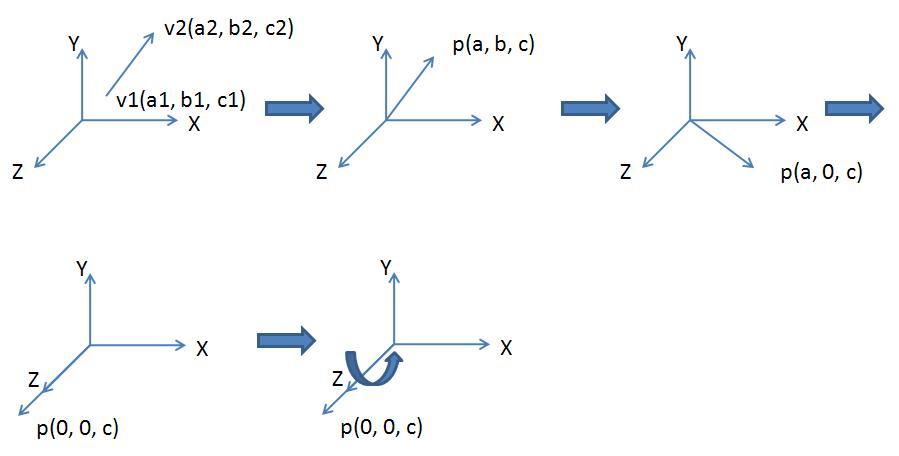步骤1是一个平移操作，将v1v2平移至原点，对应的矩阵为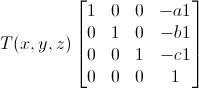步骤2是一个旋转操作，将p(p = v2 -v1)旋转至XOZ平面，步骤3也是一个旋转操作，将p旋转至与Z轴重合，这两个操作对应的图如下。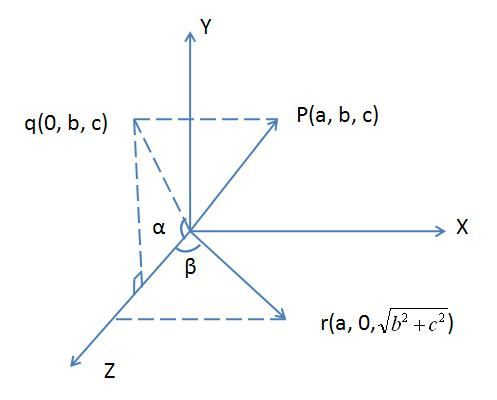做点p在平面YOZ上的投影点q。再过q做Z轴垂线，则r是p绕X轴旋转所得，且旋转角度为α，且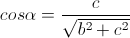,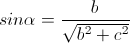于是旋转矩阵为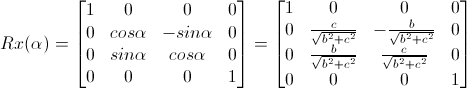现在将r绕Y轴旋转至与Z轴重合，旋转的角度为-beta（方向为顺时针），且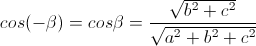,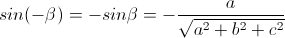于是得到旋转矩阵为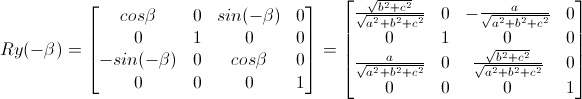最后是绕Z轴旋转，对应的矩阵如下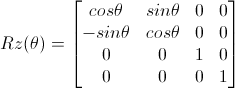如果旋转轴是过原点的，那么第一步和最后一步的平移操作可以省略，也就是把中间五个矩阵连乘起来，再转置一下，得到下面的绕任意轴旋转的矩阵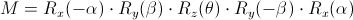即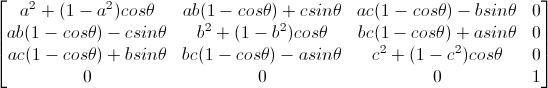对应的函数代码如下1 void RotateArbitraryAxis(D3DXMATRIX* pOut, D3DXVECTOR3* axis, float theta)
2 {
3     D3DXVec3Normalize(axis, axis);
4     float u = axis->x;
5     float v = axis->y;
6     float w = axis->z;
7
8     pOut->m = cosf(theta) + (u * u) * (1 - cosf(theta));
9     pOut->m = u * v * (1 - cosf(theta)) + w * sinf(theta);
10     pOut->m = u * w * (1 - cosf(theta)) - v * sinf(theta);
11     pOut->m = 0;
12
13     pOut->m = u * v * (1 - cosf(theta)) - w * sinf(theta);
14     pOut->m = cosf(theta) + v * v * (1 - cosf(theta));
15     pOut->m = w * v * (1 - cosf(theta)) + u * sinf(theta);
16     pOut->m = 0;
17
18     pOut->m = u * w * (1 - cosf(theta)) + v * sinf(theta);
19     pOut->m = v * w * (1 - cosf(theta)) - u * sinf(theta);
20     pOut->m = cosf(theta) + w * w * (1 - cosf(theta));
21     pOut->m = 0;
22
23     pOut->m = 0;
24     pOut->m = 0;
25     pOut->m = 0;
26     pOut->m = 1;如果旋转轴是不过原点的，那么第一步和最后一步就不能省略，将所有七个矩阵连乘起来，得到如下变换矩阵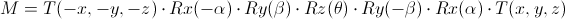对应如下这个超长的矩阵，在这里(u, v, w) = (a2, b2, c2) - (a1, b1, c1)，且是单位向量，a, b, c分别表示(a1, b1, c1)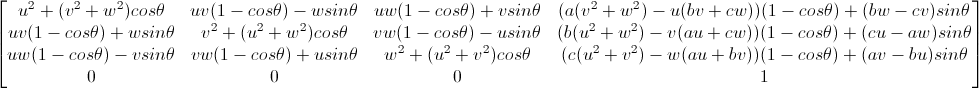将上面的过程写成函数，该函数接受四个参数，第一个参数是一个输出参数，用来保存得到的旋转矩阵，第二个和第三个参数是旋转轴的两个端点，最后一个参数是旋转角度θ，注意，在函数中我们已经将上面的矩阵转置了，因为上面是按照列向量计算的。1 void RotateArbitraryLine(D3DXMATRIX* pOut, D3DXVECTOR3* v1, D3DXVECTOR3* v2, float theta)
2 {
3     float a = v1->x;
4     float b = v1->y;
5     float c = v1->z;
6
7     D3DXVECTOR3 p = *v2 - *v1;
8     D3DXVec3Normalize(&p, &p);
9     float u = p.x;
10     float v = p.y;
11     float w = p.z;
12
13     float uu = u * u;
14     float uv = u * v;
15     float uw = u * w;
16     float vv = v * v;
17     float vw = v * w;
18     float ww = w * w;
19     float au = a * u;
20     float av = a * v;
21     float aw = a * w;
22     float bu = b * u;
23     float bv = b * v;
24     float bw = b * w;
25     float cu = c * u;
26     float cv = c * v;
27     float cw = c * w;
28
29     float costheta = cosf(theta);
30     float sintheta = sinf(theta);
31
32     pOut->m = uu + (vv + ww) * costheta;
33     pOut->m = uv * (1 - costheta) + w * sintheta;
34     pOut->m = uw * (1 - costheta) - v * sintheta;
35     pOut->m = 0;
36
37     pOut->m = uv * (1 - costheta) - w * sintheta;
38     pOut->m = vv + (uu + ww) * costheta;
39     pOut->m = vw * (1 - costheta) + u * sintheta;
40     pOut->m = 0;
41
42     pOut->m = uw * (1 - costheta) + v * sintheta;
43     pOut->m = vw * (1 - costheta) - u * sintheta;
44     pOut->m = ww + (uu + vv) * costheta;
45     pOut->m = 0;
46
47     pOut->m = (a * (vv + ww) - u * (bv + cw)) * (1 - costheta) + (bw - cv) * sintheta;
48     pOut->m = (b * (uu + ww) - v * (au + cw)) * (1 - costheta) + (cu - aw) * sintheta;
49     pOut->m = (c * (uu + vv) - w * (au + bv)) * (1 - costheta) + (av - bu) * sintheta;
50     pOut->m = 1;
51 }展开全文• [img=https://img-bbs.csdn.net/upload/201710/22/1508657472_592502.jpg][/img] 如图，不用path
• 下面是一个简单的原点旋转变换的图。 关于图形变换我们关注的都是怎么得到变换之后的坐标，而对于变换后的坐标，很多教材上都只有一个简略的结果，并不会给出详细的推导过程。今天学习旋转变换的时候，对怎么...
文章目录齐次坐标[^3]二维旋转变换三维旋转变换绕 z 轴旋转绕 x 轴旋转绕 y 轴旋转总结例子
齐次坐标1
齐次坐标表示法就是用 n+1 维向量表示一个 n 维向量。
n 维空间中的点的位置向量用非齐次坐标表示时，$(P_1,P_2,…, P_n)$ 具有 n 个坐标分量，并且是唯一的。如果用齐次坐标表示时，该向量有 n+1 个坐标分量，$(hP_1, hP_2,…, hP_n, h)$ 并且是不唯一的。2
通常都使 h=1。如果 h≠0，就可以用除齐次坐标的各分量，这一方法称之为齐次坐标的规范化。经过规范化后的齐次坐标就是唯一的。
h = 0 时，该点表示一无穷远点3。三元组 (0, 0, 0) 不表示任何点。原点表示为 (0, 0, 1)
如二维点 (x,y) 的齐次坐标表示为 $(h_x,h_y,h)$ ，则 $(h_1x,h_1y,h_1) ， (h_2x,h_2y,h_2) ，…， (h_mx,h_my,h_m)$ 都是表示二维空间中的同一个点三维空间中的坐标点的齐次坐标可表示为 (x,y,1) 。
应用齐次坐标可以有效地用矩阵运算把二维、三维甚至更高维空间中点集从一个坐标系转换到另一个坐标系中。
二维齐次坐标变换矩阵的形式为
$T_{2D} = \begin{bmatrix} a & d & g \\ b & e & h \\ c & f & i \\ \end{bmatrix}$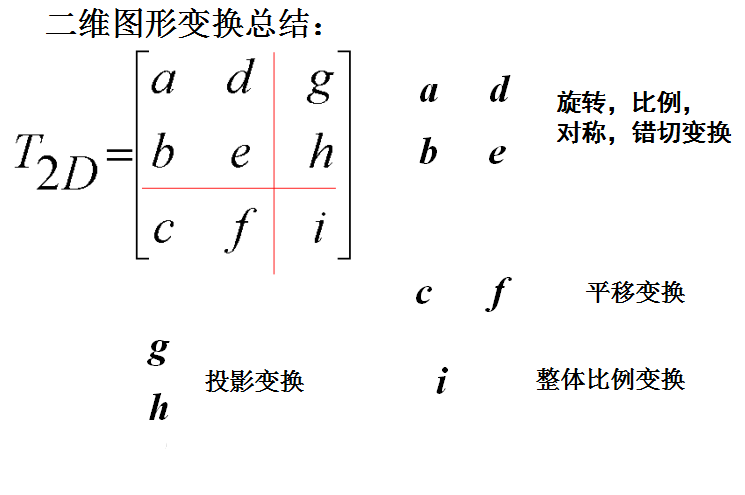三维齐次坐标变换矩阵的形式为
$T_{3D} = \begin{bmatrix} a_{11} & a_{12} & a_{13} & a_{14} \\ a_{21} & a_{22} & a_{23} & a_{24} \\ a_{31} & a_{32} & a_{33} & a_{34} \\ a_{41} & a_{42} & a_{43} & a_{44} \\ \end{bmatrix}$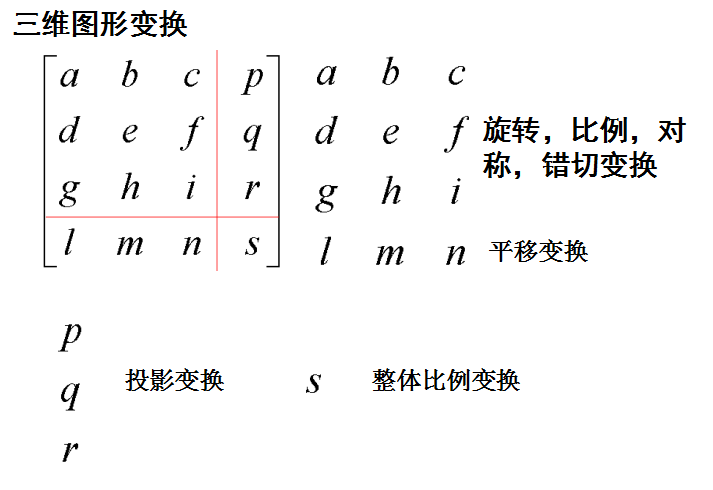二维旋转变换
下面是一个简单的绕原点旋转变换的图。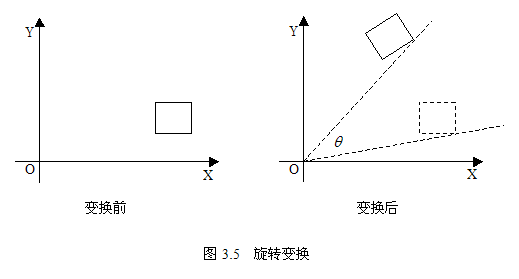本文我们关注的是怎么得到变换之后的坐标，而对于变换后的坐标，很多教材上都只有一个简略的结果，并不会给出详细的推导过程。今天学习旋转变换的时候，对怎么得出变换后的坐标产生了疑惑，花了几分钟才想明白，特此记录一下。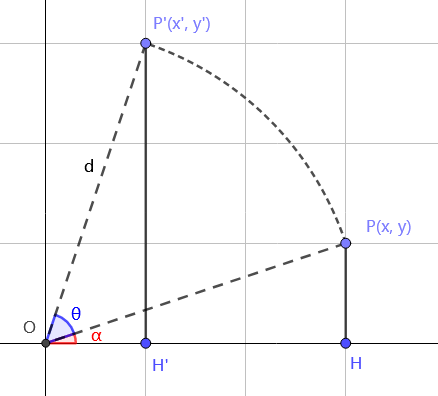我们不妨设变换前图形上任意一点的坐标为 $P(x, y)$，变换后图形上对应点坐标为 $P'(x', y')$ 。
对于旋转变换来说，我们不妨设旋转角度为 θ，即 $\angle POP' = \theta$。我们现在就来推导一下 $x'$ 和 $y'$ 关于 x, y 的表示法。（应该还有更好的证法）
由旋转的性质易知 $OP = OP' = \sqrt{x^2 + y^2} = d$
不妨设 $\angle POx = \alpha$，有 $sin\alpha = \dfrac{y}{d}$， $cos\alpha = \dfrac{x}{d}$。又有下面这两个方程：
$sin(\alpha + \theta) = \dfrac{y'}{d} \newline cos(\alpha + \theta) = \space \space \dfrac{x'}{d}$
把这两个方程解出来就可以得到 x’ 和 y’ 的值了（把 sin(a+θ)，cos(a+θ) 拆开后，sin a 和 cos a 都已知，把 d 乘过去就行，这里打方程不方便就不敲出具体拆解过程了）：
$x' = xcos\theta - ysin\theta \newline y' = xsin\theta + ycos\theta \newline$
因此有如下推导
$P' = [x'\space\space y'\space\space 1]=[xcos\theta - ysin\theta \space\space xsin\theta + ycos\theta \space\space 1]=[x\space\space y \space\space 1]\begin{bmatrix} cos\theta & sin\theta & 0 \\ -sin\theta & cos\theta & 0 \\ 0 & 0 & 1 \end{bmatrix}=P・R(\theta)$4
其坐标变换矩阵 $R(\theta)$ 如下
$\begin{bmatrix} cos\theta & sin\theta & 0 \\ -sin\theta & cos\theta & 0 \\ 0 & 0 & 1 \end{bmatrix}$
三维旋转变换
以下都是往逆时针方向旋转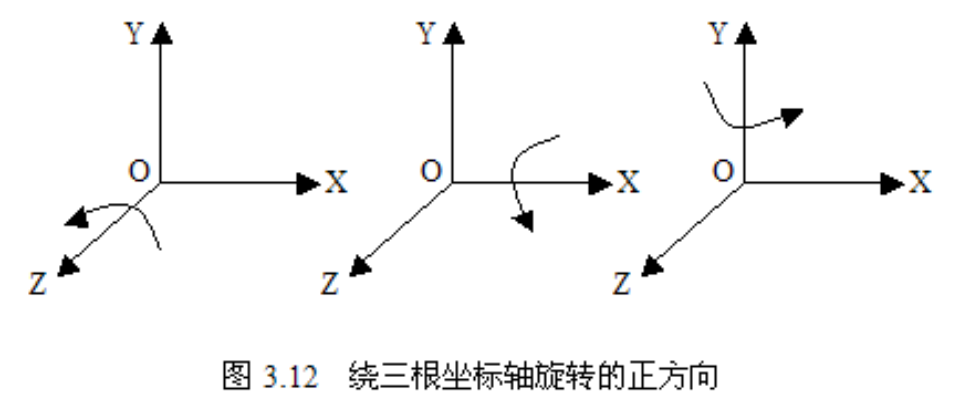分别绕三根坐标轴旋转的时候，有一个坐标是不变的，然后我们把另外两个当成二维的算就行，我们可以分别得出对应的坐标变换矩阵：
绕 z 轴旋转
$\begin{bmatrix} cos\theta & sin\theta & 0 & 0 \\ -sin\theta & cos\theta & 0 & 0 \\ 0 & 0 & 1 & 0 \\ 0 & 0 & 0 & 1 \end{bmatrix}$
绕 x 轴旋转
$\begin{bmatrix} 1 & 0 & 0 & 0 \\ 0 & cos\theta & sin\theta & 0 \\ 0 & -sin\theta & cos\theta & 0 \\ 0 & 0 & 0 & 1 \end{bmatrix}$
绕 y 轴旋转
$\begin{bmatrix} cos\theta & 0 & -sin\theta & 0 \\ 0 & 1 & 0 & 0 \\ sin\theta & 0 & cos\theta & 0 \\ 0 & 0 & 0 & 1 \end{bmatrix}$
如果说要记得话，一个简单的记法是绕哪个轴旋转哪个轴上就没有 cos sin 之类的。
总结
这个总结可以不看，现在我也看不懂了。
我们不难发现核心部分基本和二维都是差不多的，除了绕 y 轴旋转的那个 sinθ 的正负号反了。那么我们有没有想过为什么就它是反的呢？一个歪理如下：因为核心部分$\begin{bmatrix} cos\theta & sin\theta \\ -sin\theta & cos\theta \\ \end{bmatrix}$被分开了 😃以下结论对初始点在两轴正方向成立，对旋转角度也有一定要求：

对于绕 z 轴旋转，观察到 x 一直在减小
对于绕 x 轴旋转，观察到 y 一直在减小
对于绕 y 轴旋转，观察到 z 一直在减小

而坐标变换矩阵中，谁减小，负号就在谁的 sinθ 那里。
例子
例：设三维空间中有一条任意直线，它由直线上一点 Q 和沿直线方向的单位方向向量 n 确定。Q 点坐标为 $(x_0, y_0, z_0)$，直线向量 n = $[n_1, n_2, n_3]$ ，|n| = $\sqrt{{n_1}^2+{n_2}^2+{n_3}^2}$ = 1。求绕这条直线旋转 $\theta$ 角的旋转变换矩阵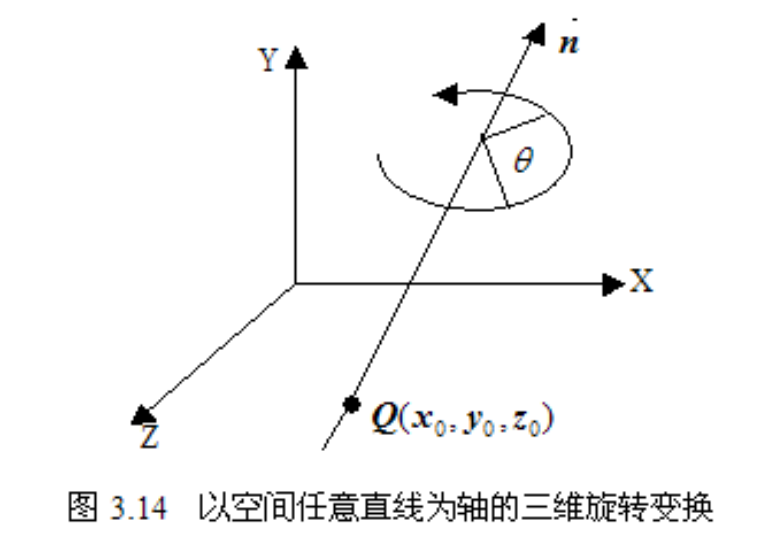实现该变换可以先做平移变换，使旋转轴成为通过坐标原点的一条直线，然后做绕通过坐标原点的旋转轴旋转 $\theta$ 角的旋转变换，最后再做平移变换，即开始所作平移变换的逆变换，使旋转轴平移回到原来的位置。
过坐标原点的任意直线为旋转轴的旋转变换可分为五步实现：

做绕 X 轴旋转 $\alpha$ 角的变换，使旋转轴落在 Y=0 上。
做绕 Y 轴旋转 $\beta$ 角的变换，使旋转轴与 Z 轴重合。
做绕 Z 轴旋转 $\theta$ 角的旋转变换。
做第 2 步的逆变换，即做绕 Y 轴 -$\alpha$ 旋转变换。
做第 1 步的逆变换，即做绕 X 轴 -$\beta$ 旋转变换。

我们不妨假设由向量 ON 表示直线向量 n = $[n_1, n_2, n_3]$，其中 O 为原点，N 坐标为 $(n_1, n_2, n_3)$。对题中直线平移到原点后，绕题中的直线旋转就相当于绕 ON 旋转。
我们要确定的值是 $\alpha$ 和 $\beta$ 这两个角，首先我们来看 $\alpha$
$\alpha$ 是绕 X 轴旋转的变换的角，使平面 OMN 落在 Y=0 上成为 $OM''N''$
$sin\alpha = \dfrac{M'M}{OM} = \dfrac{n_2}{v}$
$cos\alpha = \dfrac{M'O}{OM} = \dfrac{n_3}{v}$
其中 $OM = v = \sqrt{{n_2}^2+{n_3}^2}$，M’ 为 M 到 OZ 的垂足。
由于是绕 X 轴旋转，故其旋转矩阵为
$R_x(\alpha) = \begin{bmatrix} 1 & 0 & 0 & 0 \\ 0 & cos\alpha& sin\alpha& 0 \\ 0 & -sin\alpha& cos\alpha & 0 \\ 0 & 0 & 0 & 1 \end{bmatrix} = \begin{bmatrix} 1 & 0 & 0 & 0 \\ 0 & \dfrac{n_3}{v} & \dfrac{n_2}{v} & 0 \\ 0 & -\dfrac{n_2}{v} & \dfrac{n_3}{v} & 0 \\ 0 & 0 & 0 & 1 \end{bmatrix}$
$N' = [n_1, n_2, n_3, 1] R_x(\alpha) = [n_1, 0, v, 1]$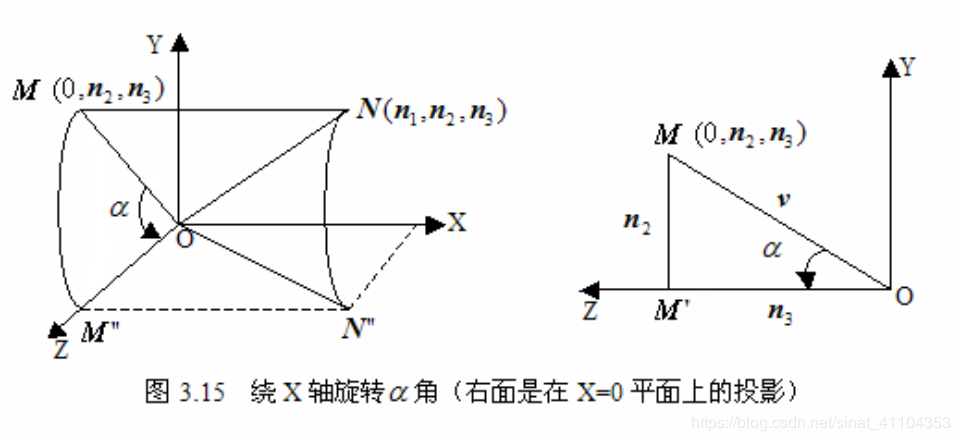然后我们再来看 $\beta$，$\beta$ 是在第一步的基础上绕 Y 轴旋转 $ON''$ 使得 $ON''$ 落在 Z 轴上
由第一步我们已经知道 $M''N'' = MN = n_1$，$d = ON'' = ON = \sqrt{{n_1}^2+{n_2}^2+{n_3}^2} = 1$，所以有
$sin\beta= -\dfrac{M''N''}{ON''} = -\dfrac{n_1}{d}=-n_1$5
$cos\beta= \dfrac{M''O}{ON''} = \dfrac{\sqrt{d^2-{n_1}^2}}{d}=\sqrt{d^2-{n_1}^2}=\sqrt{n_2^2+n_3^2}=v$
由于 $\beta$ 是绕 Y 轴旋转，故其旋转矩阵为
$R_y(\beta) = \begin{bmatrix} cos\beta & 0 & -sin\beta& 0 \\ 0 & 1 & 0 & 0 \\ sin\beta& 0 & cos\beta& 0 \\ 0 & 0 & 0 & 1 \end{bmatrix} = \begin{bmatrix} v & 0 & n_1 & 0 \\ 0 & 1 & 0 & 0 \\ -n_1 & 0 & v & 0 \\ 0 & 0 & 0 & 1 \end{bmatrix}$
$N'' = [n_1, 0, v, 1]R_y(\beta)=[0,0,1,1]$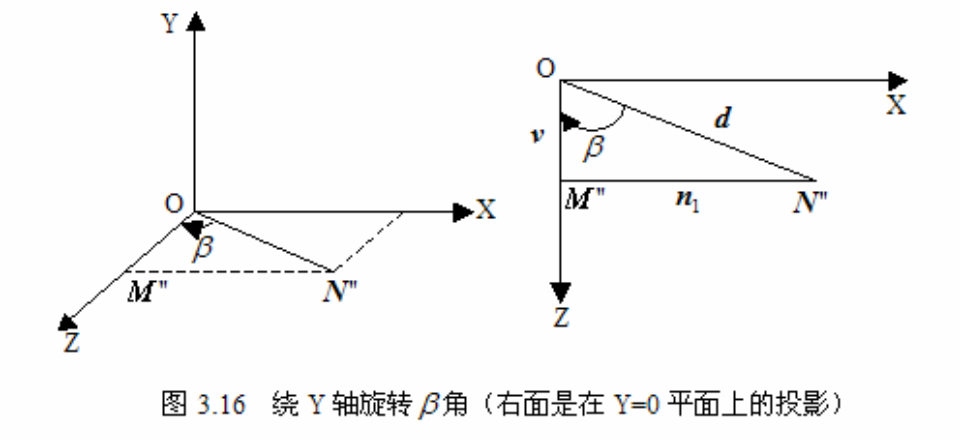又做绕 Z 轴旋转 θ 角的旋转变换。故旋转矩阵为
$R_z(\theta)=\begin{bmatrix} cos\theta & sin\theta & 0 & 0 \\ -sin\theta & cos\theta & 0 & 0 \\ 0 & 0 & 1 & 0 \\ 0 & 0 & 0 & 1 \end{bmatrix}$
回到 5 个步骤

做绕 X 轴旋转 $\alpha$ 角的变换，使旋转轴落在 Y=0 上。
做绕 Y 轴旋转 $\beta$ 角的变换，使旋转轴与 Z 轴重合。
做绕 Z 轴旋转 $\theta$ 角的旋转变换。
做第 2 步的逆变换，即做绕 Y 轴 -$\alpha$ 旋转变换。
做第 1 步的逆变换，即做绕 X 轴 -$\beta$ 旋转变换。

五个步骤用旋转矩阵表示出来如下：
$R(\theta)=R_x(\alpha)・R_y(\beta)・R_z(\theta)・R_y(-\beta) ・R_x(-\alpha) =$
$\begin{bmatrix} 1 & 0 & 0 & 0 \\ 0 & \dfrac{n_3}{v} & \dfrac{n_2}{v} & 0 \\ 0 & -\dfrac{n_2}{v} & \dfrac{n_3}{v} & 0 \\ 0 & 0 & 0 & 1 \end{bmatrix}$ ・$\begin{bmatrix} v & 0 & n_1 & 0 \\ 0 & 1 & 0 & 0 \\ -n_1 & 0 & v & 0 \\ 0 & 0 & 0 & 1 \end{bmatrix}$ ・$\begin{bmatrix} cos\theta & sin\theta & 0 & 0 \\ -sin\theta & cos\theta & 0 & 0 \\ 0 & 0 & 1 & 0 \\ 0 & 0 & 0 & 1 \end{bmatrix}$ ・$\begin{bmatrix} v & 0 & -n_1 & 0 \\ 0 & 1 & 0 & 0 \\ n_1 & 0 & v & 0 \\ 0 & 0 & 0 & 1 \end{bmatrix}$ ・$\begin{bmatrix} 1 & 0 & 0 & 0 \\ 0 & \dfrac{n_3}{v} & -\dfrac{n_2}{v} & 0 \\ 0 & \dfrac{n_2}{v} & \dfrac{n_3}{v} & 0 \\ 0 & 0 & 0 & 1 \end{bmatrix}=$
$\begin{bmatrix} n_1^2+(1-n_1^2)cos\theta & n_1n_2(1-cos\theta)+n_3sin\theta & n_1n_3(1-cos\theta)-n_2sin\theta & 0 \\ n_1n_2(1-cos\theta)-n_3sin\theta & n_2^2+(1-n_2^2)cos\theta & n_2n_3(1-cos\theta)+n_1sin\theta & 0 \\ n_1n_3(1-cos\theta)+n_2sin\theta & n_2n_3(1-cos\theta)-n_1sin\theta & n_3^2+(1-n_3^2)cos\theta & 0 \\ 0 & 0 & 0 & 1 \end{bmatrix}$

齐次坐标可让包括无穷远点的点坐标以有限坐标表示 ↩︎

[计算机图形学 第四版]: 齐次坐标（homogeneous coordinate）这一术语在数学中用来指出笛卡尔方程的表达效果。当笛卡尔点 (x, y) 转换成齐次坐标 $(x_h, y_h, h)$ 时，包含 x 和 y 的方程 f(x, y) = 0 变成了三个参数 $x_h, y_h 和 h$ 的齐次方程。这恰好说明，假如三个参数均被各自乘上值 v 后的值替换，那么 v 可以从方程中作为因子提取出来。 ↩︎

[维基百科，齐次坐标]:  一条通过原点 (0, 0) 的线之方程可写作 nx+my = 0，其中 n 及 m 不能同时为 0。以参数表示，则能写成 x=mt, y=-nt。令 Z=1/t，则线上的点之笛卡尔坐标可写作 (m/Z, -n/Z)。在齐次坐标下，则写成 (m, -n, Z)。当 t 趋向无限大，亦即点远离原点时，Z 会趋近于 0，而该点的齐次坐标则会变成 (m, -n, 0)。因此，可定义 (m, -n, 0) 为对应 nx+my=0 这条线之方向的无穷远点之齐次坐标。因为欧式平面上的每条线都会与透过原点的某一条线平行，且因为平行线会有相同的无穷远点，欧式平面每条线上的无穷远点都有其齐次坐标。 ↩︎

[维基百科，齐次坐标]: 有些作者会使用不同的符号表示齐次坐标，以与笛卡尔坐标相区别，如以冒号代替逗号，如以 (x:y:z) 代替 (x, y, z)，以强调该坐标有着比例的性质。亦有以方括号代替括弧，如以 [x, y, z] 来强调有多个坐标表示同一个点。有些作者则会同时使用冒号与方括号，如 [x:y:z]。 ↩︎

这里 $sin\beta$ 是负的是因为 $\beta$ 是顺时针旋转，我们默认逆时针方向为正方向。 ↩︎


展开全文• 2.任意一点旋转变换(由键盘输入旋转角度和旋转中心) 编译器：vs2013 1 #include "stdafx.h" 2 #include<stdio.h> 3 #include"graphics.h" 4 #include<stdlib.h> 5 #include<m...

1.编码实现绕原点的旋转变换(旋转角度由键盘输入),
2.绕任意一点的旋转变换(由键盘输入旋转角度和旋转中心)
编译器：vs2013

1 #include "stdafx.h"
2 #include<stdio.h>
3 #include"graphics.h"
4 #include<stdlib.h>
5 #include<math.h>
6
7 #define PI 3.14159
8
9 void spin00(int a[], double angle);
10 void spinxy(int a[], double angle, int x, int y);
11
12 int main()
13 {
14     int gdriver=DETECT, gmove;
15     double angle;
16     int a = { 100, 100, 150, 150, 250, 150, 250, 0, 350, 150, 450, 150, 500, 200, 450, 250, 350, 250, 250, 400, 250, 250, 150, 250, 100, 300, 100, 100 };
17
18     /*printf("please input the angle:\n");
19     scanf_s("%lf", &angle);
20
21     initgraph(&gdriver, &gmove, "");
22
23     drawpoly(14, a);
24
25     spin00(a, angle);*/
26
27     int x, y;
28
29     printf("please input the angle:\n");
30     scanf_s("%lf", &angle);
31     printf("please input the point:\n");
32     scanf_s("%d%d", &x, &y);
33
34     initgraph(&gdriver, &gmove, "");
35
36     spinxy(a, angle, x, y);
37
38     system("pause");
39
40     closegraph();
41
42     return 0;
43 }
44
45 //绕原点旋转变换
46 void spin00(int a[], double angle)
47 {
48     int i,b;
49
50     for (i = 0; i < 28; i = i + 2)
51     {
52         b[i] = a[i] * cos(angle / 180.0*PI) - a[i + 1] * sin(angle / 180.0*PI);
53         b[i + 1] = a[i] * sin(angle / 180.0*PI) + a[i + 1] * cos(angle / 180.0*PI);
54     }
55
56     drawpoly(14, b);
57 }
58
59 //绕（x，y）旋转变换
60 void spinxy(int a[], double angle, int x, int y)
61 {
62     int i, b,t;
63
64     for (i = 0; i < 28; i = i + 2)
65     {
66         b[i] = a[i] - x;
67         b[i + 1] = a[i + 1] - y;
68         t = b[i];
69         b[i] = b[i] * cos(angle / 180.0*PI) - b[i + 1] * sin(angle / 180.0*PI);
70         b[i + 1] = t * sin(angle / 180.0*PI) + b[i + 1] * cos(angle / 180.0*PI);
71         b[i] = b[i] + x;
72         b[i + 1] = b[i + 1] + y;
73     }
74
75     drawpoly(14, b);
76 }

小结：在第二题时需要注意b[i]在操作过程中被改变，需要设置新变量来存储旧值

转载于:https://www.cnblogs.com/cdp1591652208/p/6873776.html
展开全文• 以制作“三角形平面上任意一点旋转的动画”为例，具体的操作步骤如下： 步骤一 打开教学课件制作软件几何画板，使用左侧“线段工具”绘制任意三角形ABC，然后使用“点工具”在三角形外绘制任意一点O，双击点O，...
作为21世纪的动态几何工具，几何画板完美地实现了动态教学，可以用它给学生们演示图形的运动过程和状态，比如可以实现某平面图形围绕一个点做旋转动画，那么具体要怎么做呢？
以制作“三角形绕平面上任意一点旋转的动画”为例，具体的操作步骤如下：
步骤一 打开教学课件制作软件几何画板，使用左侧“线段工具”绘制任意三角形ABC，然后使用“点工具”在三角形外绘制任意一点O，双击点O，标记为旋转中心；步骤二 点击上方的“数据”菜单，在下拉菜单选择“新建参数”命令，在弹出的新建参数对话框将单位改为角度，然后点击“确定”；步骤三 选中上步新建的角度参数，然后点击上方的“变换”菜单，在下拉菜单选择“标记角度”命令，如下图所示；步骤四 选中需要旋转的三角形ABC，点击上方的“变换”菜单，在下拉菜单选择“旋转”命令，角度就使用标记的角度，然后点击“确定”；步骤五 选中角度参数，点击上方的“编辑”菜单，在下拉菜单选择“操作类按钮”——“动画”，在弹出的动画按钮对话框点击“确定”。鼠标点击参数动画按钮，三角形绕点旋转的动画就开了。当然手动改变参数的角度，也可以有动画出现。以上给大家讲解了在几何画板中制作三角形围绕点旋转的动画，主要运用了几何画板旋转功能和制作动画功能。几何画板变换菜单中的“旋转”变换，可以将图像绕某点旋转需要的角度，如果你还是很陌生，可以自己动手试试！
转载于:https://blog.51cto.com/14241007/2397520
展开全文• 那么如何推导出二维图形绕一点旋转的公式呢？我在这里就将其推导过程简要的说明一下。 其实推导过程比较简单，首先我们来看一幅图，看看如何推导出二维图形绕原点进行旋转的公式。 上图画的比较粗略，不过能...
• 把一个平面图形绕着平面内一点O转动一个角度，叫做图形的旋转。点O为选中中心，转动的角为旋转角，如果图形上的一点P经过旋转变为P’，则这两个点为这个旋转的对应点。 中心对称 如图： 把一个图形绕着一个点旋转...数学 基础 教程
• 那么如何推导出二维图形绕一点旋转的公式呢？我在这里就将其推导过程简要的说明一下。 其实推导过程比较简单，首先我们来看一幅图，看看如何推导出二维图形绕原点进行旋转的公式。 上图画的比较粗略，不过能...
• 二维平面上，一点绕任意中心点旋转任意角度，所使用函数如下： function rotateRectByCenterPos(pos, centerPos, angle) { var originX = centerPos["x"]; var originY = centerPos["y"]; angle = angle * Math....javascript
• 对称图形有很多分类，例如轴对称图形：如果一个图形沿着一条直线对折后两部分完全重合，这样的图形叫做轴对称图形。如果一个图形绕一点旋转180度，旋转后的图形能和原图形完全重合，那么这个图形...nagios 微软 css 数据可视化
• 旋转变换是由一个图形改变为另一个图形，在改变过程中，原图上所有的点都一个固定的点换同一方向，转动同一个角度。 欧氏几何中的一种重要变换 目录 1简介 2性质 简介 编辑 简称旋转。欧氏几何中的一种...
• 平行四边形 概述 平行四边形是初二最难的一章，对中考的影响较大 目前仍有考试做不出来的风险（不小），仍需提高绝对水平 ...一个图形绕着某一点旋转$180^{\circ}$...
• 二维图形可看作由许多直线连接而成，或是许多小直线段逼近而成。一个线段有两个端点构成，故一个图形可以看成点的集合，因此变换均可通过对...旋转变换是将一物体旋转点沿圆弧路径移动旋转并定位（默认情况下...
• GIS坐标旋转陈玉进 李泉 （南京跬步科技有限公司http://www.creable.cn ） 在地理信息系统中有对地图进行旋转显示的需求，例如GPS导航系统...以坐标原点为圆心的旋转图形一点P(x,y)原点逆时针旋转a角后得到新的c
• 点p0p0坐标原点逆时针方向旋转θθ角度得到点p1p1.  从①②可以得到旋转变换的矩阵公式：  以任意图形中心点为坐标原点旋转原理： 从上图可知以任意图形中心点为坐标原点旋转我们需...
• 以制作“三角形平面上任意一点旋转的动画”为例，具体的操作步骤如下： 步骤一 打开几何画板，使用左侧“线段工具”绘制任意三角形ABC，然后使用“点工具”在三角形外绘制任意一点O，双击点O，...
• 在OPENGL ES的几乎大部分教程里，如果你稍微仔细一点会发现一个一直隐藏的问题：他们的选择都是基于GlRotate()这个函数来实现的，当你控制鼠标或者触屏来旋转物体的时候，初始时如果只一个方向旋转是正确的，但是...opengles unity3d opengl
• 实验二：直线的光栅化算法 DDA Bresenham 实验三：圆的光栅化算法 编程实现两种中点画圆算法，第2种算法利用二阶差分方法；...任意选一点为中心，将其经过平移到达坐标系原点的位置，然后原点进行旋转，最后将...matlab 光栅 算法 几何变换
• 一点P沿动射线OP以等速率运动的同时，该射线又以等角速度点O旋转，点P的轨迹称为“阿基米德螺线”。 阿基米德螺线的笛卡尔坐标方程式为： r=10*(1+t) x=r*cos(t * 360) y=r*sin(t *360) 编写如下的HTML...
• //正方形自己中心旋转角度α，同时放大cosα+sinα //给出画一个齿的数据，旋转20次，画出一个齿轮： //四条斜靠在坐标轴四面上的直线，沿轴移动，形成星形图案。 //平移、放缩、旋转、正平行投影（三视图）、一点...
• 正视图:(yz)俯视图:(xy)侧视图:(xz)1.2 正轴测投影:如图:咱们希望按照这个平面对物体进行平行投影:首先,先把物体及投影面沿着y轴顺时针旋转,在x轴逆时针旋转θ角,使投影面与z轴重合,变换矩阵如下:最后做Z方向的正...
• 要求风车的三个叶片以及中间的黄色三角形（在其所在平面上）一起着中心一点不停旋转，且将此动画渲染出来。 实现通过键盘对动画的交互控制，包括切换旋转方向、增大旋转速度以及减小旋转速度。 设计按钮和菜单...opengl c++ glut
• 坐标变换矩阵是一个3*3的矩阵，用来对图形进行坐标变化，将原来的坐标点转移到新的坐标点，因为一个图片是有点阵和每一点上的颜色信息组成的，所以对坐标的变换，就是对每一点进行搬移形成新的图片。具体的说图形的...图像处理 缩放 变换 矩阵
• 1.坐标系 (1).Unity 世界空间坐标系为左手系，Y正向向上的左手系。这一点在需要从3ds max里导入模型到Unity时需要注意，3ds max使用的... Unity 的坐标变换为：着x轴旋转90度,直接使用四元数变换如下： 1 pu...
• 这一篇讲述变换（Transformation） 仿射...旋转是让一个刚体绕一点运动的变换。缩放变换是让物体的形状扩大与减小，并且在各个方向上的缩放因子都相同。平移变换将每个点沿着指定的方向移动常量距离。错切对于给定轴线gtk matrix callback signal
• 对于每个touchesBegan（），我将在屏幕中央创建一个新的平面框，该框与相机对齐，并在touchesMoved（）上，我将使用touch位置附加到要绘制到的路径上我将CAShapeLayer用作新创建的几何图形的漫反射材料。 简单的！ ...